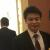求个简单的纯数字java加密算法，可逆

0public class StringHelper{

public static String decode(String strIn) {
if (isEmpty(strIn))
return "";
String strTemp = "";
for (int i = 0; i < strIn.length(); i++) {
char charTemp = strIn.charAt(i);
switch (charTemp) {
case 124: // '~'
String strTemp2 = strIn.substring(i + 1, i + 3);
strTemp = strTemp + (char) Integer.parseInt(strTemp2, 16);
i += 2;
break;

case 94: // '^'
String strTemp3 = strIn.substring(i + 1, i + 5);
strTemp = strTemp + (char) Integer.parseInt(strTemp3, 16);
i += 4;
break;

default:
strTemp = strTemp + charTemp;
break;
}
}

return strTemp;
}

public static String encode(String strIn) {
if (isEmpty(strIn))
return "";
String strOut = "";
for (int i = 0; i < strIn.length(); i++) {
int iTemp = strIn.charAt(i);
if (iTemp > 255) {
String strTemp2 = Integer.toString(iTemp, 16);
for (int iTemp2 = strTemp2.length(); iTemp2 < 4; iTemp2++)
strTemp2 = "0" + strTemp2;

strOut = strOut + "^" + strTemp2;
} else {
if (iTemp < 48 || iTemp > 57 && iTemp < 65 || iTemp > 90
&& iTemp < 97 || iTemp > 122) {
String strTemp2 = Integer.toString(iTemp, 16);
for (int iTemp2 = strTemp2.length(); iTemp2 < 2; iTemp2++)
strTemp2 = "0" + strTemp2;

strOut = strOut + "|" + strTemp2;
} else {
strOut = strOut + strIn.charAt(i);
}
}
}

return strOut;
}

}